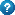Electrical energy is called the energy contained in moving electrons (or other charged particles or ions).
Electrical energy can also be stored, (electrostatics) and under appropriate circumstances will result in motion of electrons (e.g. lightning, thunder).In metals there are free electrons which are moving randomly from atom to atom.

If at the ends of a metallic conductor a potential difference (voltage) is applied e.g. from a battery, then the free electrons forced to move from the point with low potential to the one with high potential in other words from the negative pole of the battery to the positive one. This flow of electrons is called electric current.

Electric current is therefore the directed movement of electrons.

How the electrical energy used by an electrical appliance is calculated?